Latest SSC jobs   »   Quantitative Aptitude For SSC CGL :...

# Quantitative Aptitude For SSC CGL : 4th February 2020 for Simple & Compound interest

Q1. Priya lent Rs. 10,000 partly at the rate of 4 percent and partly at the rate of 5 percent per annum, at simple interest for purchased a wristwatch. The total interest after 2 years is Rs. 880. The sum of money lent at each of the above rates is to be divided in the ratio:
(a) 4: 5
(b) 3: 2
(c) 5: 4
(d) 2: 3

Q2. Simple interest on a certain amount is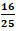of the principal. If the rate of interest and time (in years) be equal, then time, for which the principal is lent out, is: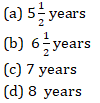Q3. At a certain rate of simple interest an amount of Rs. 4000 becomes Rs. 5240 in 10 years. If the rate of interest had been 7% more, the same amount would have become-
(a) Rs. 8250
(b) Rs. 8040
(c) Rs. 8530
(d) None of these

Q4. Gopal borrows Rs. 8000 from a bank at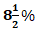compound interest. At the end of every year, he pays Rs. 2100 as part repayment of loan and interest. How much does he still owes to the bank after two such installments?
(a) Rs. 5039.3
(b) Rs. 5099.3
(c) Rs. 4769.3
(d) Rs. 4995.3

Q5. Rishi lends Rs. 4500 to Neeraj and a certain sum to Shashank at the same time at 13% annual simple interest. If after 5 years Rishi received interest of Rs. 5694 from Neeraj and Shashank, the sum lent to Shashank is
(a) Rs. 6250
(b) Rs. 6562
(c) Rs. 65220
(d) Rs. 4260

Q6. Arun loses Rs. 653.10 yearly when the annual rate of interest falls from 13.5% to 10.35%. His capital (in rupees) is
(a) 8866.67
(b) 8682.33
(c) 6866.33
(d) 8869.33

Q7. Shashank borrows Rs. 4000 at 20% compound rate of interest. At the end of each year he pays back Rs. 1800. How much amount should he pay at the starting of the Forth year to clear all his dues?
(a) 2160
(b) 2530
(c) 2460
(d) 1800

Q8. Dev lent out a certain sum on simple interest and the same sum on compound interest at a certain rate of interest per annum. He noticed that the ratio between the difference of compound interest and simple interest of 3 years and that of 2 years is 25 : 8. The rate of interest per annum is: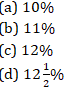Q9. Divide Rs. 51867 in two parts so that the simple interest on the first when deposited for one year at 11% per annum and that on the second when deposited for two years at 9.25% per annum in a bank are the same.
(a) Rs. 26851 and 26538
(b) Rs. 12681 and Rs. 39186
(c) Rs. 26781 and Rs. 25086
(d) Rs. 26536 and Rs. 25331

Q10. A sum of money was lent at simple interest at 11% per annum for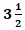years and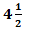years respectively. If the difference in interest for two periods was Rs. 412.50, then find the sum?
(a) Rs. 3570
(b) Rs. 7530
(c) Rs. 5730
(d) Rs. 3750

Solutions: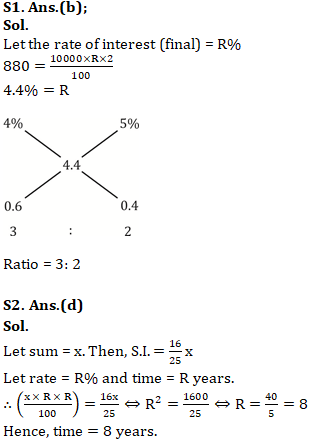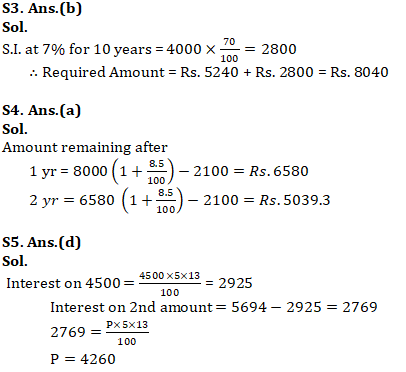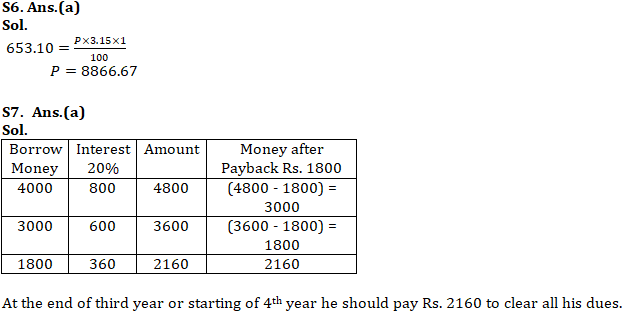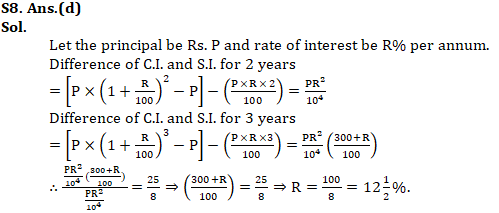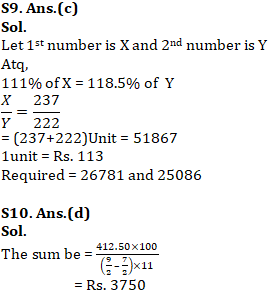#### Congratulations!General Awareness & Science Capsule PDFIncorrect details? Fill the form again here

General Awareness & Science Capsule PDF

Thank You, Your details have been submitted we will get back to you.
•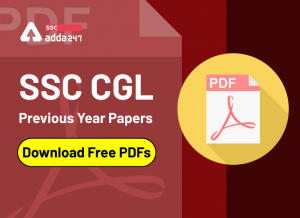SSC CGL Previous Year Question Paper Wit...
•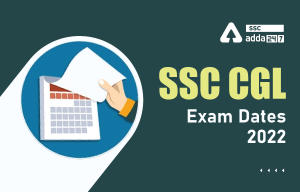SSC CGL Exam Date 2022, Check Complete E...
•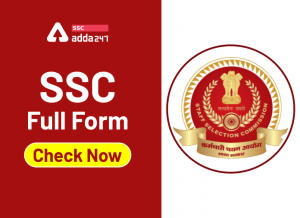SSC Full Form, How Staff Selection Commi...
•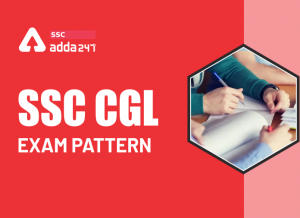SSC CGL Exam Pattern 2022, Revised Exam ...
•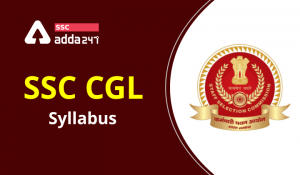SSC CGL Syllabus 2022 Out, Detailed Syll...
•SSC CGL 2022 Notification PDF Out, Apply...